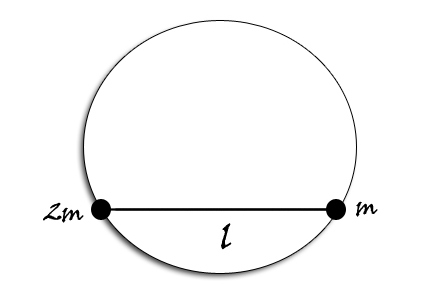# Constrained, uneven dumbbellTwo beads of mass $\displaystyle 2m$ and $\displaystyle m$, connected by a rod of length $\displaystyle\ell$ and of negligible mass, are free to move in a smooth vertical circular wire frame of radius $\displaystyle\ell$ as shown.

Calculate the velocity (in $\displaystyle m/s$) that should be given to the mass $\displaystyle 2m$ (when the rod is in horizontal position) in counter-clockwise direction so that the rod just becomes vertical.

Details and Assumptions:
$\bullet$ $\displaystyle m = 250g$
$\bullet$ $\displaystyle \ell = 25cm$
$\bullet$ $\displaystyle g = 9.8 m/s^2$

Note: This problem appeared in our AITS (All India Test Series) - 6

×

Problem Loading...

Note Loading...

Set Loading...